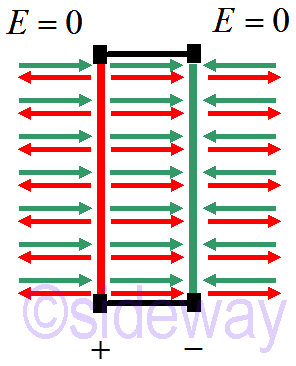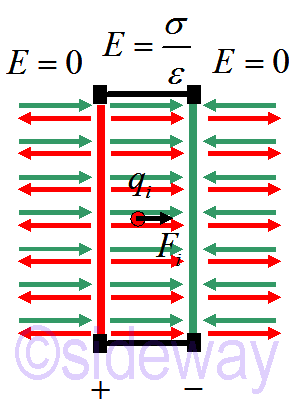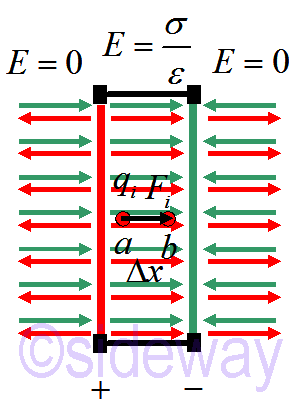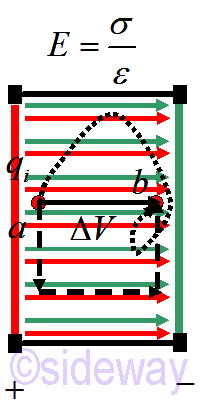output.to from Sideway
Draft for Information Only

# Content

`Electric Potential Potential Difference  Non-uniform Electric Field Path of Potential Difference Source and Reference`

# Electric Potential

For two fixed opposite charged plates, the electric field due to plates is as followingIf a charged particle is placed in the capacitor, an induced force will drive the charged particle to move.The eletric pontential energy acting on the charge particle 𝑞𝑖 is `𝑈ele= ∑𝑗𝑈𝑖𝑗= ∑𝑗14𝜋𝜀0𝑞𝑖𝑞𝑗𝑟𝑖𝑗` The electric potential is defined as the potential or the ability to have potential energy if a test charge enters the system. In other words, the electric potential can be expressed as ``` 𝑉≡𝑈ele𝑞=JoulesCoulomb=[Volts]```

## Potential Difference

When the charge particle is moved from 𝑎 to 𝑏 as followingthe change in potential energy of the system is ```∆𝑈=−𝑊internal=−∫𝑞𝐸∙𝑑𝑟=−𝑞∫𝐸𝑥𝑑𝑥 ⇒∆𝑈=−𝑞𝐸∆𝑥 ``` In which the systems tends to lower their potential energy by moving the charge particle from a higher potential energy position to a lower potential energy position. In other words, the potential difference drive the charge particle to move from one position to another. ```∆𝑈=−𝑞𝐸∆𝑥≡𝑞∆𝑉⇒∆𝑉=∆𝑈𝑞=−𝐸∆𝑥 𝑉=[Volt]=JouleCoulomb ∆𝑈/𝑞=−𝐸∆𝑥≡∆𝑉⇒𝐸=−∆𝑈/𝑞∆𝑥=−∆𝑉/∆𝑥 |𝐸|=JouleCoulomb-meter=NewtonCoulomb=VoltMeter ``` An electron-Volt, 𝑒𝑉 is the energy required to move a charge particle, 𝑞=1𝑒=1.6×10−19𝐶 through 1𝑉. That is 1.6×10−19𝐽=1𝑒𝑉

### Non-uniform Electric Field

For a uniform electric field, i.e. 𝐸∥𝑥, ∆𝑉=−𝐸∆𝑥. And for a uniform electric field pointing in any direction. `∆𝑉=−(𝐸𝑥∆𝑥+𝐸𝑦∆𝑦+𝐸𝑧∆𝑧)≡−𝐸∙𝑑𝑟` If the electric field 𝐸 is not uniform, but varies in space. The potential difference can be determined by a line integral like stepping along a path. At each step, use component of electric field parallel to the step direction. `∆𝑉=−𝑓∑𝑖𝐸∙𝑑𝑙=−𝑓∫𝑖𝐸∙𝑑𝑙` The electric field can therefore be expressed as `𝐸=−𝑑𝑉𝑑𝑥=−∂∂𝑥𝑉𝑥+∂∂𝑦𝑉𝑦+∂∂𝑧𝑉𝑧=−∇𝑉`

## Path of Potential DifferenceSince electricity is a conservative force the potential difference ∆𝑉 is independent of the path taken. For example, in a simple uniform electric field `∆𝑉=−𝑓∑𝑖𝐸∙𝑑𝑙=−𝑓∑𝑖𝐸𝑥𝑑𝑙𝑥+𝑓∑𝑖𝐸𝑦𝑑𝑙𝑦+𝑓∑𝑖𝐸𝑧𝑑𝑙𝑧=𝑉𝑓−𝑉𝑖`

## Source and Reference

ID: 191200302 Last Updated: 12/3/2019 Revision: 0Home 5

Management

HBR 3

Information

Recreation

Culture

Chinese 1097

English 337

Computer

Hardware 154

Software

Application 207

Latex 35

Manim 203

Numeric 19

Programming

Web 285

Unicode 504

HTML 65

CSS 63

SVG 9

ASP.NET 240

OS 422

Python 64

Knowledge

Mathematics

Algebra 84

Geometry 32

Calculus 67

Engineering

Mechanical

Rigid Bodies

Statics 92

Dynamics 37

Control

Natural Sciences

Electric 27